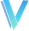•闪存
•博客
• 发言小组
• 投递新闻
• 提问博问
• 添加收藏
•文库

# .net 实现类似 excel 公式的方法

0悬赏园豆：20 [已解决问题] 解决于 2023-04-10 16:51百鸟朝凤| 菜鸟二级 | 园豆：262

0

``````using OfficeOpenXml;
using var package = new ExcelPackage();
sheet.Cells["A1"].Value = "abc";
sheet.Cells["A2"].Value = "def";
sheet.Cells["A3"].Formula = "3+(if(len(A1)+1>5,1,0)+len(A2))*20";
sheet.Calculate();
Console.WriteLine("Result: " + sheet.Cells["A3"].Text);
// Result: 65
``````

dudu | 高人七级 |园豆：32634 | 2023-03-31 10:23

@百鸟朝凤: 或者借助 Source Generators 根据公式动态生成 C# 代码

dudu | 园豆：32634 (高人七级) | 2023-03-31 10:29

@dudu: 嗯...我看了一下,好像也不太行...我现在的难点是如何快速解析这个公式, 计算要遵循优先级, 还有最后的四则运算,写的也比较吃力

@百鸟朝凤: 可以看看这篇博文 Writing a Simple Math Expression Engine in C#

dudu | 园豆：32634 (高人七级) | 2023-03-31 10:45

0

excel 公式里的LEN函数 是返回字符串的字符数，IF 函数 跟 .net 三元表达式一样，下面就是用.NET 翻译过来的excel 公式

``````string rsult = (3 +( (abc.ToString().Length > 5 ? 1 : 0) + (def.ToString().Length) )* 20).ToString();
``````

您需要登录以后才能回答，未注册用户请先注册